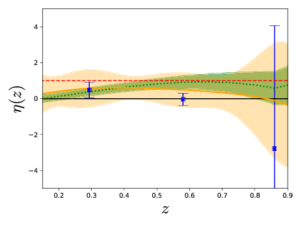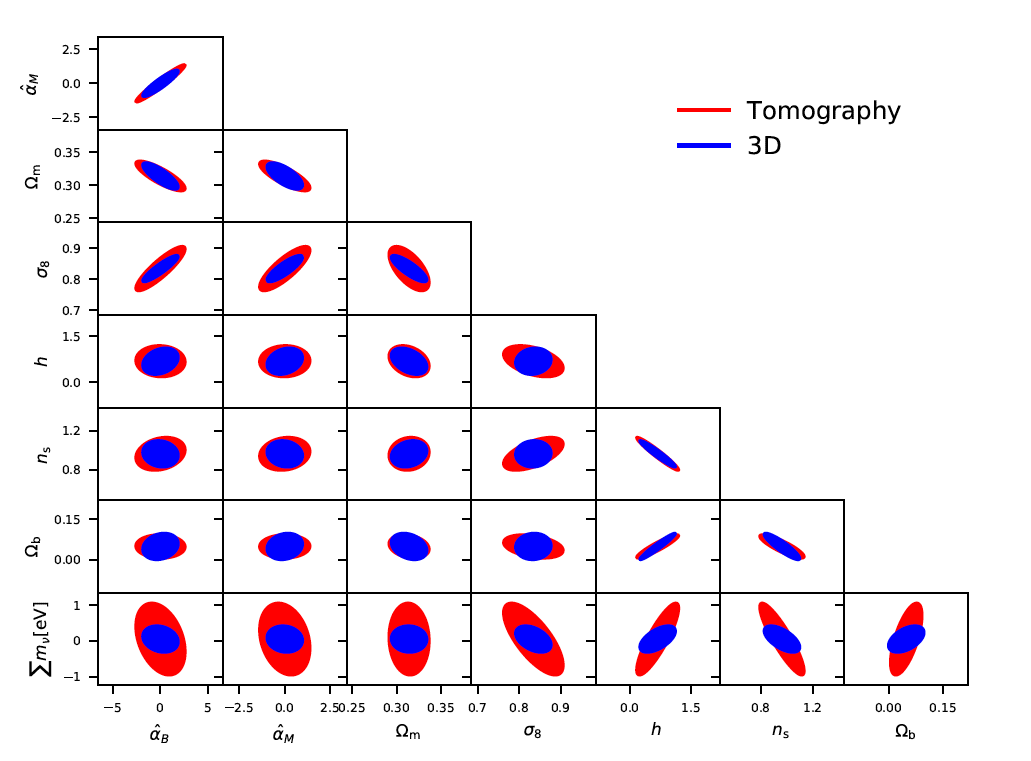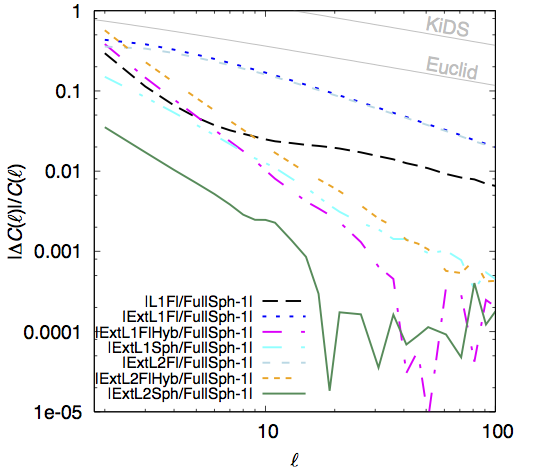## Abstract

Non-linear bias measurements require a great level of control of potential systematic effects in galaxy redshift surveys. Our goal is to demonstrate the viability of using Counts-in-Cells (CiC), a statistical measure of the galaxy distribution, as a competitive method to determine linear and higher-order galaxy bias and assess clustering systematics. We measure the galaxy bias by comparing the first four moments of the galaxy density distribution with those of the dark matter distribution. We use data from the MICE simulation to evaluate the performance of this method, and subsequently perform measurements on the public Science Verification (SV) data from the Dark Energy Survey (DES). We find that the linear bias obtained with CiC is consistent with measurements of the bias performed using galaxy-galaxy clustering, galaxy-galaxy lensing, CMB lensing, and shear+clustering measurements. Furthermore, we compute the projected (2D) non-linear bias using the expansion $\delta_{g} = \sum_{k=0}^{3} (b_{k}/k!) \delta^{k}$, finding a non-zero value for $b_2$ at the $3\sigma$ level. We also check a non-local bias model and show that the linear bias measurements are robust to the addition of new parameters. We compare our 2D results to the 3D prediction and find compatibility in the large scale regime ($>30$ Mpc $h^{-1}$)

## Abstract

In this manuscript of the habilitation à diriger des recherches (HDR), the author presents some of his work over the last ten years. The main topic of this thesis is cosmic shear, the distortion of images of distant galaxies due to weak gravitational lensing by the large-scale structure in the Universe. Cosmic shear has become a powerful probe into the nature of dark matter and the origin of the current accelerated expansion of the Universe. Over the last years, cosmic shear has evolved into a reliable and robust cosmological probe, providing measurements of the expansion history of the Universe and the growth of its structure.
I review the principles of weak gravitational lensing and show how cosmic shear is interpreted in a cosmological context. Then I give an overview of weak-lensing measurements, and present observational results from the Canada-France Hawai'i Lensing Survey (CFHTLenS), as well as the implications for cosmology. I conclude with an outlook on the various future surveys and missions, for which cosmic shear is one of the main science drivers, and discuss promising new weak cosmological lensing techniques for future observations.

## Abstract

We present a new method to estimate shear measurement bias in image simulations that significantly improves its precision with respect to the state-of-the-art methods. This method is based on measuring the shear response for individual images. We generate sheared versions of the same image to measure how the shape measurement changes with the changes in the shear, so that we obtain a shear response for each original image, as well as its additive bias. Using the exact same noise realizations for each sheared version allows us to obtain an exact estimation of its shear response. The estimated shear bias of a sample of galaxies comes from the measured averages of the shear response and individual additive bias. The precision of this method supposes an improvement with respect to previous methods since our method is not affected by shape noise. As a consequence, the method does not require shape noise cancellation for a precise estimation of shear bias. The method can be easily applied to many applications such as shear measurement validation and calibration, reducing the number of necessary simulated images by a few orders of magnitude to achieve the same precision requirements.

## Breaking degeneracies in modified gravity with higher (than 2nd) order weak-lensing statistics

### Breaking degeneracies in modified gravity with higher (than 2nd) order weak-lensing statistics

 Authors: A. Peel, V. Pettorino, C. Giocoli, J.-L. Starck, M. Baldi Journal: A&A Year: 2018 Download: ADS | arXiv

## Abstract

General relativity (GR) has been well tested up to solar system scales, but it is much less certain that standard gravity remains an accurate description on the largest, that is, cosmological, scales. Many extensions to GR have been studied that are not yet ruled out by the data, including by that of the recent direct gravitational wave detections. Degeneracies among the standard model (ΛCDM) and modified gravity (MG) models, as well as among different MG parameters, must be addressed in order to best exploit information from current and future surveys and to unveil the nature of dark energy. We propose various higher-order statistics in the weak-lensing signal as a new set of observables able to break degeneracies between massive neutrinos and MG parameters. We have tested our methodology on so-called f(R) models, which constitute a class of viable models that can explain the accelerated universal expansion by a modification of the fundamental gravitational interaction. We have explored a range of these models that still fit current observations at the background and linear level, and we show using numerical simulations that certain models which include massive neutrinos are able to mimic ΛCDM in terms of the 3D power spectrum of matter density fluctuations. We find that depending on the redshift and angular scale of observation, non-Gaussian information accessed by higher-order weak-lensing statistics can be used to break the degeneracy between f(R) models and ΛCDM. In particular, peak counts computed in aperture mass maps outperform third- and fourth-order moments.

## Model-independent reconstruction of the linear anisotropic stress

 Authors: Ana Marta Pinho,  Santiago Casas, Luca Amendola Journal: Accepted for JCAP Year: 05/2018 Download: Inspire| Arxiv

## Abstract

In this work, we use recent data on the Hubble expansion rate H(z), the quantity fσ8(z) from redshift space distortions and the statistic Eg from clustering and lensing observables to constrain in a model-independent way the linear anisotropic stress parameter η. This estimate is free of assumptions about initial conditions, bias, the abundance of dark matter and the background expansion. We denote this observable estimator as ηobs. If ηobs turns out to be different from unity, it would imply either a modification of gravity or a non-perfect fluid form of dark energy clustering at sub-horizon scales. Using three different methods to reconstruct the underlying model from data, we report the value of ηobs at three redshift values, z=0.29,0.58,0.86. Using the method of polynomial regression, we find ηobs=0.57±1.05, ηobs=0.48±0.96, and ηobs=0.11±3.21, respectively. Assuming a constant ηobs in this range, we find ηobs=0.49±0.69. We consider this method as our fiducial result, for reasons clarified in the text. The other two methods give for a constant anisotropic stress ηobs=0.15±0.27 (binning) and ηobs=0.53±0.19 (Gaussian Process). We find that all three estimates are compatible with each other within their 1σ error bars. While the polynomial regression method is compatible with standard gravity, the other two methods are in tension with it.## Testing (modified) gravity with 3D and tomographic cosmic shear

 Authors: A. Spurio Mancini, R. Reischke, V. Pettorino, B.M. Scháefer, M. Zumalacárregui Journal: Submitted to MNRAS Year: 2018 Download: ADS | arXiv

## Abstract

Cosmic shear, the weak gravitational lensing caused by the large-scale structure, is one of the primary probes to test gravity with current and future surveys. There are two main techniques to analyse a cosmic shear survey; a tomographic method, where correlations between the lensing signal in different redshift bins are used to recover redshift information, and a 3D approach, where the full redshift information is carried through the entire analysis. Here we compare the two methods, by forecasting cosmological constraints for future surveys like Euclid. We extend the 3D formalism for the first time to theories beyond the standard model, belonging to the Horndeski class. This includes the majority of universally coupled extensions to LCDM with one scalar degree of freedom in addition to the metric, which are still in agreement with current observations. Given a fixed background, the evolution of linear perturbations in Horndeski gravity is described by a set of four functions of time only. We model their time evolution assuming proportionality to the dark energy density fraction and place Fisher matrix constraints on the proportionality coefficients. We find that a 3D analysis can constrain Horndeski theories better than a tomographic one, in particular with a decrease in the errors on the Horndeski parameters of the order of 20 - 30%. This paper shows for the first time a quantitative comparison on an equal footing between Fisher matrix forecasts for both a fully 3D and a tomographic analysis of cosmic shear surveys. The increased sensitivity of the 3D formalism comes from its ability to retain information on the source redshifts along the entire analysis.

## Summary

A new paper has been put on the arXiv, led by Alessio Spurio Mancini, PhD student of CosmoStat member Valeria Pettorino in collaboration with R. Reischke, B.M. Scháefer (Heidelberg) and M. Zumalacárregui (Berkeley LBNL and Paris Saclay IPhT).
The authors investigate the performance of a 3D analysis of cosmic shear measurements vs a tomographic analysis as a probe of Horndeski theories of modified gravity, setting constraints by means of a Fisher matrix analysis on the parameters that describe the evolution of linear perturbations, using the specifications of a future Euclid-like experiment. Constraints are shown on both the modified gravity parameters and on a set of standard cosmological parameters, including the sum of neutrino masses. The analysis is restricted to angular modes ell < 1000 and k < 1 h/Mpc to avoid the deeply non-linear regime of structure growth. Below the main results of the paper.

• The signal-to-noise ratio of both a 3D analysis as well as a tomographic one is very similar.
• 3D cosmic shear provides tighter constraints than tomography for most cosmological parameters, with both methods showing very similar degeneracies.
• The gain of 3D vs tomography is particularly significant for the sum of the neutrino masses (factor 3). For the Horndeski parameters the
gain is of the order of 20 - 30 % in the errors.
•  In Horndeski theories, braiding and the effective Newton coupling parameters (\alpha_B and \alpha_M) are constrained better if the kineticity is higher.
• We investigated the impact on non-linear scales, and introduced an artificial screening scale, which pushes the deviations from General Relativity to zero below its value.  The gain when including the non-linear signal calls for the development of analytic or semi-analytic prescriptions for the treatment of non-linear scales in ΛCDM and modified gravity.## Abstract

We present cosmological results from a combined analysis of galaxy clustering and weak gravitational lensing, using 1321 deg$^2$ of $griz$ imaging data from the first year of the Dark Energy Survey (DES Y1). We combine three two-point functions: (i) the cosmic shear correlation function of 26 million source galaxies in four redshift bins, (ii) the galaxy angular autocorrelation function of 650,000 luminous red galaxies in five redshift bins, and (iii) the galaxy-shear cross-correlation of luminous red galaxy positions and source galaxy shears. To demonstrate the robustness of these results, we use independent pairs of galaxy shape, photometric redshift estimation and validation, and likelihood analysis pipelines. To prevent confirmation bias, the bulk of the analysis was carried out while blind to the true results; we describe an extensive suite of systematics checks performed and passed during this blinded phase. The data are modeled in flat $\Lambda$CDM and $w$CDM cosmologies, marginalizing over 20 nuisance parameters, varying 6 (for $\Lambda$CDM) or 7 (for $w$CDM) cosmological parameters including the neutrino mass density and including the 457 $\times$ 457 element analytic covariance matrix. We find consistent cosmological results from these three two-point functions, and from their combination obtain $S_8 \equiv \sigma_8 (\Omega_m/0.3)^{0.5} = 0.783^{+0.021}_{-0.025}$ and $\Omega_m = 0.264^{+0.032}_{-0.019}$ for $\Lambda$CDM for $w$CDM, we find $S_8 = 0.794^{+0.029}_{-0.027}$, $\Omega_m = 0.279^{+0.043}_{-0.022}$, and $w=-0.80^{+0.20}_{-0.22}$ at 68% CL. The precision of these DES Y1 results rivals that from the Planck cosmic microwave background measurements, allowing a comparison of structure in the very early and late Universe on equal terms. Although the DES Y1 best-fit values for $S_8$ and $\Omega_m$ are lower than the central values from Planck ...

## Precision calculations of the cosmic shear power spectrum projection

 Authors: M. Kilbinger, C. Heymans, M. Asgari et al. Journal: MNRAS Year: 2017 Download: ADS | arXiv

## Abstract

We compute the spherical-sky weak-lensing power spectrum of the shear and convergence. We discuss various approximations, such as flat-sky, and first- and second- order Limber equations for the projection. We find that the impact of adopting these approximations are negligible when constraining cosmological parameters from current weak lensing surveys. This is demonstrated using data from the Canada-France-Hawaii Lensing Survey (CFHTLenS). We find that the reported tension with Planck Cosmic Microwave Background (CMB) temperature anisotropy results cannot be alleviated, in contrast to the recent claim made by Kitching et al. (2016, version 1). For future large-scale surveys with unprecedented precision, we show that the spherical second-order Limber approximation will provide sufficient accuracy. In this case, the cosmic-shear power spectrum is shown to be in agreement with the full projection at the sub-percent level for l > 3, with the corresponding errors an order of magnitude below cosmic variance for all l. When computing the two-point shear correlation function, we show that the flat-sky fast Hankel transformation results in errors below two percent compared to the full spherical transformation. In the spirit of reproducible research, our numerical implementation of all approximations and the full projection are publicly available within the package nicaea at http://www.cosmostat.org/software/nicaea.

## Summary

We discuss various methods to calculate projections for weak gravitational lensing: Since lenses galaxies pick up matter inhomogeneities of the cosmic web along the line of sight while photons from the galaxies propagate through the Universe to the observer, these inhomogeneities have to be projected to a 2D observable, the cumulative shear or convergence. The full projection involves three-dimensional integrals over highly oscillating Bessel functions, and can be time-consuming to compute numerically to high accuracy. Most previous work have therefore used approximations such as the Limber approximation, that reduce the integrals to 1D, and thereby neglecting modes along the line of sight.

The authors show that these projections are more than adequate for present surveys. Sub-percent accuracy is reached for l>20, for example as shown by the pink curve, which is the ratio of the case 'ExtL1Hyb' to the full projection. The abbreviation means 'extended', corresponding to the improved approximation introduced by LoVerde & Afshordi (2008), first-order Limber, and hybrid, since this is a hybrid between flat-sky and spherical coordinates. This case has been used in most of the recent publications (e.g. for KiDS), whereas the cast 'L1Fl' (first-order Limber flat-sky) was popular for most publications since 2014.

These approximations are sufficient for the small areas of current observations coming from CFHTLenS, KiDS, and DES, and well below cosmic variance of even future surveys (the figure shows Euclid - 15,000 deg2 and Kids -1,500 deg2).The paper then discusses the second-order Limber approximation, introduced in a general framework by LoVerde & Afshordi (2008), and applied to weak lensing in the current paper. The best 2nd-order case 'ExtL2Sph' reaches sub-percent accuracy down to l=3, sufficient for all future surveys.

The paper also computes the shear correlation function in real space, and shows that those approximations have a very minor influence.

We then go on to re-compute the cosmological constraints obtained in Kilbinger et al. (2013), and find virtually no change when choosing different approximations. Only the depreciated case 'ExtL1Fl' makes a noticeable difference, which is however still well within the statistical error bars. This case shows a particular slow convergence to the full projection.

Similar results have been derived in two other recent publications, Kitching et al. (2017), and Lemos, Challinor & Efstathiou (2017).
Note however that Kitching et al. (2017) conclude that errors from projection approximations of the types we discussed here (Limber, flat sky) could make up to 11% of the error budget of future surveys. This is however assuming the worst-case scenario including the deprecated cast 'ExtL1Fl', and we do not share their conclusion, but think that for example the projection 'ExtL2Sph' is sufficient for future surveys such as LSST and Euclid.

## Abstract

DESI (Dark Energy Spectroscopic Instrument) is a Stage IV ground-based dark energy experiment that will study baryon acoustic oscillations (BAO) and the growth of structure through redshift-space distortions with a wide-area galaxy and quasar redshift survey. To trace the underlying dark matter distribution, spectroscopic targets will be selected in four classes from imaging data. We will measure luminous red galaxies up to $z=1.0$. To probe the Universe out to even higher redshift, DESI will target bright [O II] emission line galaxies up to $z=1.7$. Quasars will be targeted both as direct tracers of the underlying dark matter distribution and, at higher redshifts ($2.1 < z < 3.5$), for the Ly-$\alpha$ forest absorption features in their spectra, which will be used to trace the distribution of neutral hydrogen. When moonlight prevents efficient observations of the faint targets of the baseline survey, DESI will conduct a magnitude-limited Bright Galaxy Survey comprising approximately 10 million galaxies with a median $z\approx 0.2$. In total, more than 30 million galaxy and quasar redshifts will be obtained to measure the BAO feature and determine the matter power spectrum, including redshift space distortions.

## Abstract

DESI (Dark Energy Spectropic Instrument) is a Stage IV ground-based dark energy experiment that will study baryon acoustic oscillations and the growth of structure through redshift-space distortions with a wide-area galaxy and quasar redshift survey. The DESI instrument is a robotically-actuated, fiber-fed spectrograph capable of taking up to 5,000 simultaneous spectra over a wavelength range from 360 nm to 980 nm. The fibers feed ten three-arm spectrographs with resolution

$R= λ/Δλ$

between 2000 and 5500, depending on wavelength. The DESI instrument will be used to conduct a five-year survey designed to cover 14,000 deg

$^2$

. This powerful instrument will be installed at prime focus on the 4-m Mayall telescope in Kitt Peak, Arizona, along with a new optical corrector, which will provide a three-degree diameter field of view. The DESI collaboration will also deliver a spectroscopic pipeline and data management system to reduce and archive all data for eventual public use.